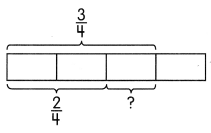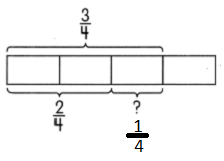# Math in Focus Grade 2 Chapter 12 Practice 3 Answer Key Adding and Subtracting Like Fractions

Practice the problems of Math in Focus Grade 2 Workbook Answer Key Chapter 12 Practice 3 Adding and Subtracting Like Fractions to score better marks in the exam.

## Math in Focus Grade 2 Chapter 12 Practice 3 Answer Key Adding and Subtracting Like Fractions

Write the fraction for the shaded parts.

Question 1.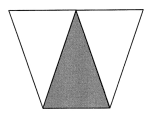Explanation:
Given,
Picture has 1 shaded part in 3.

Question 2.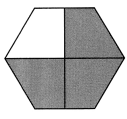Explanation:
Given,
Picture has 3 shaded parts in 4.

Question 3.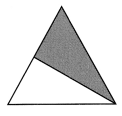Explanation:
Given,
Picture has 1 shaded part in 2.

Shade the parts to show the sum.

Example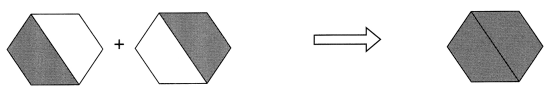Question 4.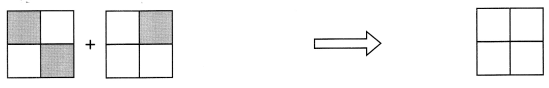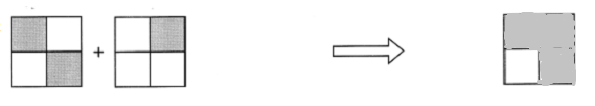Given,
Picture has 3 shaded parts in 4

Question 5.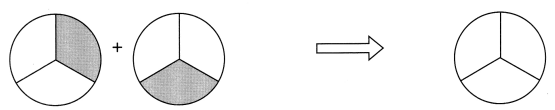Given,
Picture has 2 shaded parts in 3.
So, the fraction is 2/3

Shade the parts to show the sum.

Question 6.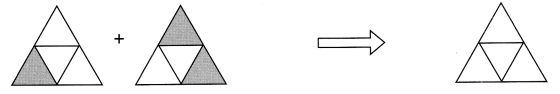Given,
Picture has 3 shaded parts in 4.
So, the fraction is 3/4

Question 7.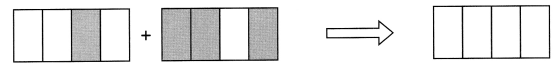Picture has 3 shaded parts in 4.
So, the fraction is 3/4

Example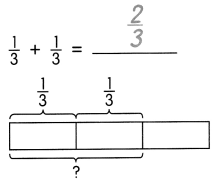Question 8.
$$\frac{1}{4}$$ + $$\frac{2}{4}$$ = ___________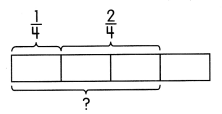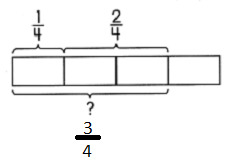Question 9.
$$\frac{1}{2}$$ + $$\frac{1}{2}$$ = ____________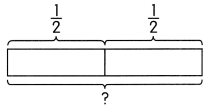Answer: 1 / 2 + 1 / 2 = 2 / 2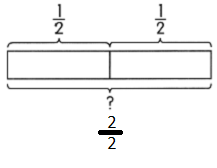Question 10.
$$\frac{1}{3}$$ + ___________ = 1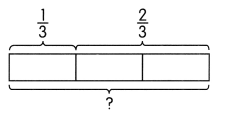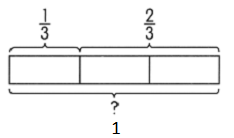Shade the parts to show the difference.

Example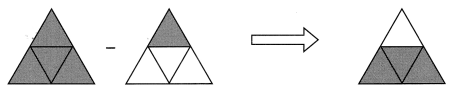Question 11.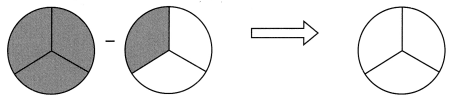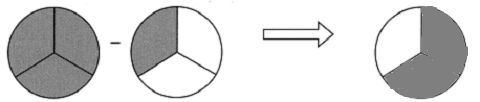Question 12.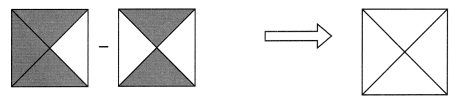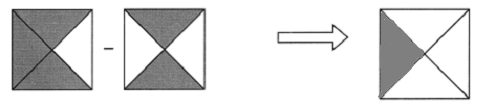Question 13.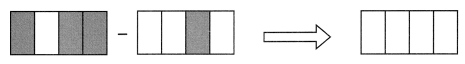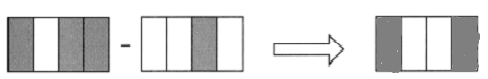Question 14.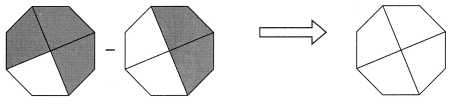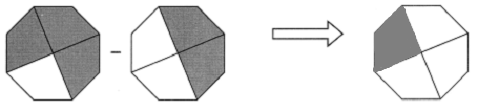Example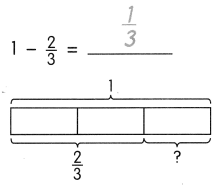Question 15.
$$\frac{3}{4}$$ – $$\frac{1}{4}$$ = ____________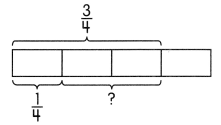Answer: 3 / 4 – 1 / 4 = 2 / 4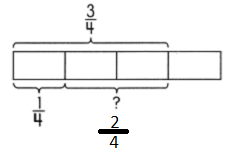Question 16.
$$\frac{2}{3}$$ – $$\frac{1}{3}$$ = ____________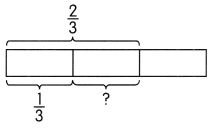Answer: 2 / 3 – 1 / 3 = 1 / 3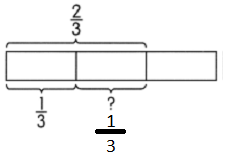Question 17.
1 – _____________ = $$\frac{1}{4}$$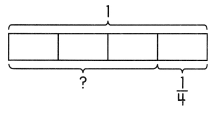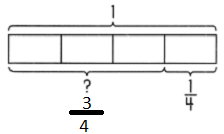$$\frac{3}{4}$$ – $$\frac{2}{4}$$ = _____________